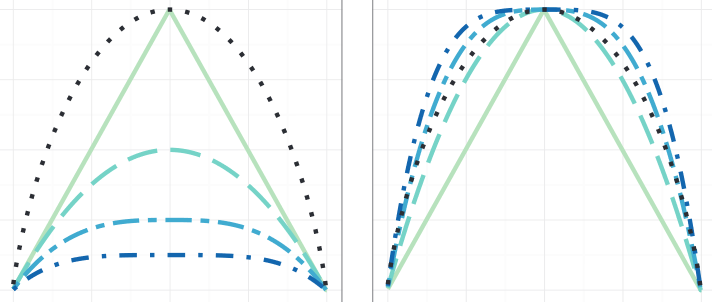# Norm-induced Entropies for Decision Forests### Abstract

The entropy measurement function is a central element of decision forest induction. The Shannon entropy and other generalized entropies such as the Renyi and Tsallis entropy are designed to fulfill the Khinchin-Shannon axioms. Whereas these axioms are appropriate for physical systems, they do not necessarily model well the artificial system of decision forest induction. In this paper, we show that when omitting two of the four axioms, every norm induces an entropy function. The remaining two axioms are sufficient to describe the requirements for an entropy function in the decision forest context. Furthermore, we introduce and analyze the p-norm-induced entropy, show relations to existing entropies and the relation to various heuristics that are commonly used for decision forest training. In experiments with classification, regression and the recently introduced Hough forests, we show how the discrete and differential form of the new entropy can be used for forest induction and how the functions can simply be fine-tuned. The experiments indicate that the impact of the entropy function is limited, however can be a simple and useful post-processing step for optimizing decision forests for high performance applications.

Type